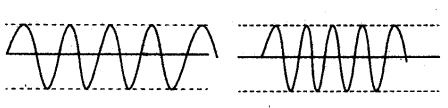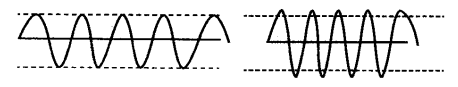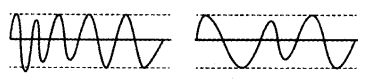# Represent graphically two separate diagrams in each case: (a) Two sound waves having same amplitude but different frequencies

Represent graphically two separate diagrams in each case:
(a) Two sound waves having same amplitude but different frequencies.
(b) Two sound waves with same frequency and different amplitudes.
© Two sound waves having varying amplitudes
and different wavelengths.

(a) Two sound waves having same amplitude but different frequencies.(b) Two sound waves with same frequency and different amplitudes.© Two sound waves having varying amplitudes and different wavelengths.